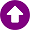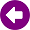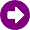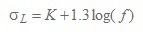﻿ Calculation method > ITU-R Rec. P.1546

# ITU-R REC. P.1546

Navegación:  Calculation method > ITU-R Rec. P.1546

# ITU-R REC. P.1546DESCRIPTION

The ITU-R Recommendation P.1546 , “Method for point-to-area predictions for terrestrial services in the frequency range 30 MHz to 3000 MHz”, describes a method for point-to-area radio propagation predictions for terrestrial services in the frequency range 30 MHz to 3 000 MHz. It is intended for use on tropospheric radio circuits over land paths, sea paths and/or mixed land-sea paths between 1‑1000 km length for effective transmitting antenna heights less than 3000 m . The method is based on interpolation/extrapolation from empirically derived field-strength curves as functions of distance, antenna height, frequency and percentage time. The calculation procedure also includes corrections to the results obtained from this interpolation/extrapolation to account for terrain clearance and terminal clutter obstructions.

The method converges with the Okumura-Hata model results under conditions for which their applications ranges overlap.

The model is restricted to:

f: 30 to 3000 [MHz]

h1: = 3000 [m]

h2: > 1 (land), > 3 m (sea) [m]

d: 1 to 1000 [km]

The method is briefly described in the next Section. The calculations are not described in full detail, because of their complexity and the worldwide availability of ITU-R Recommendations. The complete details of calculations are available in .

DEVELOPMENT

The following input data are required:

Frequency f [MHz].

Distance d [km].

Transmitting/base antenna height h1[m]. Its value depends on the type and length of the path. For sea paths, it is the antenna height above sea level. For land paths, the effective height of the transmitting/base antenna, heff, is defined as its height in metres above the average ground level between distances of 3 and 15 km from the transmitting/base antenna in the direction of the receiving/mobile antenna. For land paths longer than 15 km, h1 = heff. Detailed calculations are presented in  for shorter land paths.

Receiver/mobile antenna height h2[m]. It is the antenna height above the local terrain.

Percentage of time T%. The procedures deliver the field strength exceeded for this time percentage.

Percentage of locations L%. The procedures deliver the field strength exceeded for this locations percentage.

Terrain data: Land, cold sea and warm sea areas along the path must be identified. Detailed altitude information is needed to calculate h1 as well as the “terrain clearance angle”, if the corresponding correction is to be used.

Clutter data: Urban and suburban areas must be identified if the corresponding correction is to be used.

Field strength versus distance curves are given in  for the following conditions:

Distance: 1 to 1000 km.

Frequency: 100, 600 and 2000 MHz.

1%, 10% and 50% of time.

Land, cold and warm sea.

Transmitting/base antenna height h1: Eight normalized values from 10 to 1200 meters.

Receiving/mobile antenna height h2 equals the representative clutter height.

50% of locations.

A total of 24 figures are included in  with these plots: 8 for each reference frequency. Of them, there are 2 for 50% of time (land and sea) and 3 for the other two percentages of time (land, warm and cold sea). Within each figure, eight curves are plotted, corresponding to each normalized value of h1.

If the path comprises sections over land and sea, the procedure for mixed paths must be applied.

An interpolation/extrapolation procedure is given in  to calculate the field strength for non-standard values of h1. Different calculations are provided for the cases of h1 between 10 m and 3000 m, 0 m < h1 < 10 m and negatives values of h1.

If frequency of interest is different from the three normalized values, an interpolation / extrapolation procedure should be used. Starting from the data at 100 and 600 MHz, field strength values for frequencies between 30 and 600 MHz are obtained. Similarly, field strength values for frequencies between 600 MHz and 3000 MHz can be calculated from the data at 600 and 2000 MHz.

If time percentage of interest is different from the normalized values, an interpolation procedure is provided to calculate the field strength for any time percentage between 1% and 50%. Extrapolation outside this margin is not allowed.

All the procedures mentioned so far have in common the use of different curves to obtain interpolation/extrapolation values. Once all of them have been applied, the resultant field strength value corresponds to the required distance, frequency, h1 height, time percentage and land/sea path. The field strength vs. distance curves are no longer used. Some corrections are then applied.

If local information of the terrain around the receiving/mobile antenna is available, a terrain clearance angle can be calculated and used to take into account the signal diffraction in nearby obstacles.

A method is provided to calculate in detail the field strength due to tropospheric scattering, as the previous steps may not take full account of this propagation mechanism. If this method produces a result higher than the previous value, the new one must be used.

The curves have been calculated for receiving/mobile station height, h2, aqual to hR, the representative clutter height. If this is not the case, a correction for the actual value h2, can be used.

The location variability is modeled with a normal distribution whose mean value is the field strength calculated in the previous steps. The standard deviation σL (dB) can be estimated with the following equation :(1)

where:

K = 1.2, for receivers with antennas below clutter height in urban or suburban environments for mobile systems with omnidirectional antennas at car-roof height

K = 1.0, for receivers with rooftop antennas near the clutter height

K = 0.5, for receivers in rural areas

f: required frequency (MHz)

For broadcasting systems in VHF and UHF σL = 5.5 dB is usually considered for digital systems, whereas σL = 8.3 dB and 9.5 dB are used for analogue systems at 100 and 600 MHz, respectively.

This correction procedure can be used for percentages between 1% and 99%.

Some combinations of corrections could lead to field strength values that exceed maximum values that can be physically achieved. The resulting field strength must be checked to the maximum given by free space propagation, with some enhancement for small percentages of time in sea paths.

REFERENCES

 ITU-R Recommendation P.1546-4, "Method for point-to-area predictions for terrestrial services in the frequency range 30 MHz to 3000 MHz", ITU, Geneva, Switzerland, 2009.